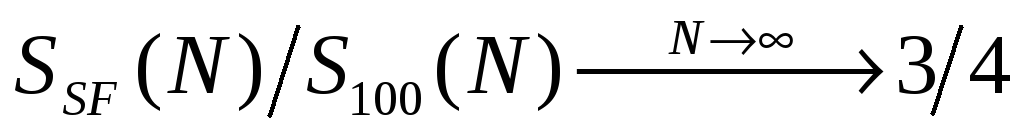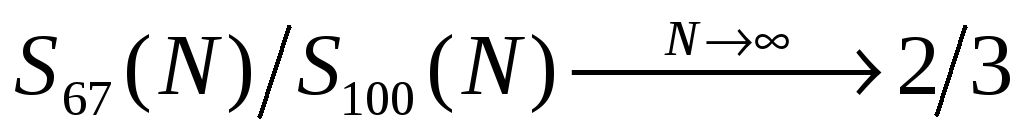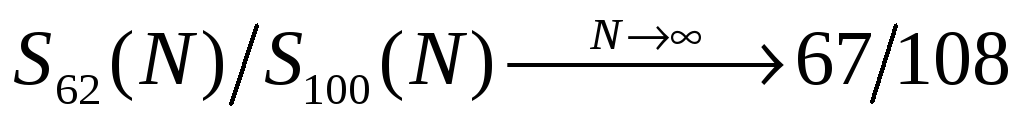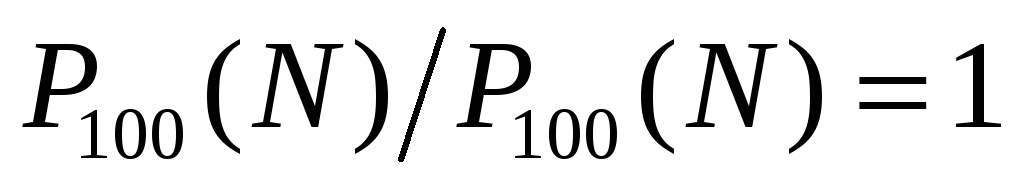# The Magnetic Tower of Hanoi

 Figure 12: Duration ratio curves for the "SF" Algorithm, the "67" Algorithm, and for the "62" Algorithm. The limits are 3/4, 2/3, and 67/108 respectively. We can see now the effect of "freedom" on solution "efficiency":(21A)(21B)(21C) The (fully) Colored MToH has the lowest efficiency defined as 1 [], the SemiFree MToH is next with efficiency of 3/4, and for the (completely) Free MToH we have found two algorithms – "67" and "62" with efficiencies of 2/3 and 67/108 respectively. The three "duration-ratio" curves are shown in Figure 12. Next is a set of recursive equations showing again the "starring" role of the number "3". Download 2.78 Mb.Share with your friends: